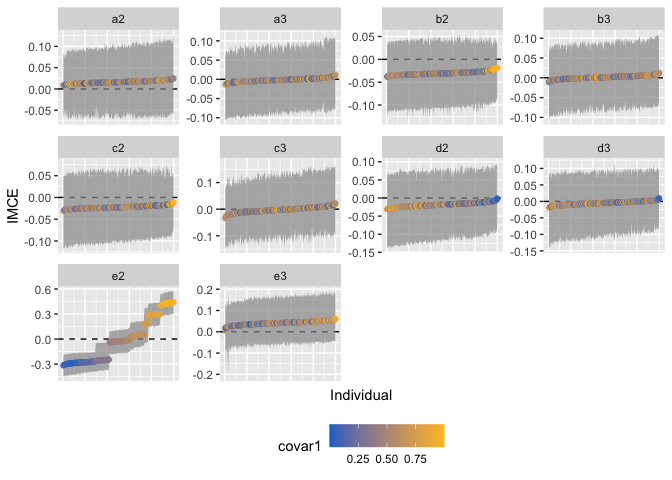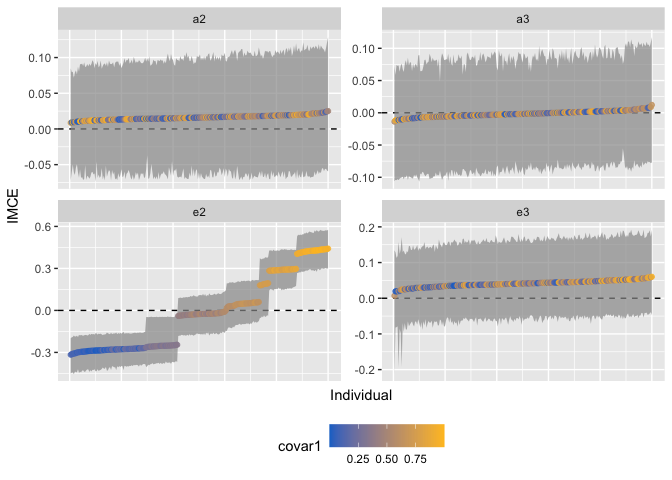# Heterogeneous effects analysis of conjoint experiments

This vignette provides a brief example of how to estimate heterogeneous effects in conjoint experiments, using .

We first simulate a basic conjoint design for the purpose of illustration. Suppose we conducted a conjoint experiment on 250 individuals, where each individual makes 5 discrete choices between two profiles. Each profile has five attributes (A-E), and we also record two covariates for each subject. The resulting data contains 2500 observations.

subjects <- 250
rounds <- 5
profiles <- 2
obs <- subjects*rounds*profiles

fake_data <- data.frame(A = sample(c("a1","a2","a3"), obs, replace = TRUE),
B = sample(c("b1","b2","b3"), obs, replace = TRUE),
C = sample(c("c1","c2","c3"), obs, replace = TRUE),
D = sample(c("d1","d2","d3"), obs, replace = TRUE),
E = sample(c("e1","e2","e3"), obs, replace = TRUE),

covar1 = rep(runif(subjects, 0 ,1),
each = rounds),
covar2 = rep(sample(c(1,0),
subjects,
replace = TRUE),
each = rounds),

id1 = rep(1:subjects, each=rounds),
stringsAsFactors = TRUE)

fake_data$Y <- ifelse(fake_data$E == "e2",
rbinom(obs, 1, fake_data\$covar1),
sample(c(0,1), obs, replace = TRUE))

## Train a probit BART model on conjoint data

To estimate a conjoint model on this data we will use the cjbart() function, which uses Bayesian Additive Regression Trees (BART) to approximate the relationships between the outcome (a binary choice), the randomized attribute-levels, and the included covariates. The result is a modified pbart model, which we will use to estimate heterogeneity in the treatment effects:

cj_model <- cjbart::cjbart(data = fake_data,
Y = "Y",
id = "id1")

To generate heterogeneity estimates at the observation- and individual-level, we use the IMCE() function. We pass in both the original experimental data, and the conjoint model generated in the last step. At this point, we also declare the names of the conjoint attribute variables (attribs) and the reference category we want to use for each attribute when calculating the marginal component effects (ref_levels). The vector of reference levels must be of the same length as attribs, with the level at position i corresponding to the attrib[i]:

het_effects <- cjbart::IMCE(data = fake_data,
model = cj_model,
attribs = c("A","B","C","D","E"),
ref_levels = c("a1","b1","c1","d1","e1"),
cores = 1)
#> Calculating OMCEs for attribute: A [1/5]
#> Calculating OMCEs for attribute: B [2/5]
#> Calculating OMCEs for attribute: C [3/5]
#> Calculating OMCEs for attribute: D [4/5]
#> Calculating OMCEs for attribute: E [5/5]
#> Calculating IMCEs

IMCE() returns an object of class “cjbart”, which contains the individual-level marginal component effects (IMCEs), matrices reflecting the upper and lower values of each estimate’s confidence interval RESPECTIVELY, and optionally the underlying observation-level marginal component effects (OMCEs) when keep_omces = TRUE.

It is likely that users will want to compare the conjoint estimation strategy to other estimation strategies, such as logistic regression models. We can recover the average marginal component effect (AMCE) for the BART model using the summary() command:

summary(het_effects)
#> Summary table of individual marginal component effects (IMCEs)
#>    Level         AMCE         Min.         Max.     Std.Dev
#> 1     a3 -0.001682914 -0.013719811  0.012178397 0.004532568
#> 2     a2  0.016041171  0.008654298  0.025002265 0.003198577
#> 3     b2 -0.030487056 -0.037984467 -0.018283045 0.003663894
#> 4     b3  0.001263259 -0.010873941  0.012479534 0.004027871
#> 5     c2 -0.022320564 -0.031114924 -0.010818911 0.003542140
#> 6     c3 -0.002632401 -0.033442283  0.021785030 0.009990552
#> 7     d3 -0.005179425 -0.019379780  0.009785969 0.005296785
#> 8     d2 -0.018312734 -0.031660234 -0.001397325 0.005577953
#> 9     e2 -0.022688508 -0.316252500  0.441821599 0.254006897
#> 10    e3  0.040823524  0.006988970  0.060000778 0.008766715

We can plot the IMCEs, color coding the points by some covariate value, using the in-built plot() function:

plot(het_effects, covar = "covar1")To aid presentation, the plot function can restrict which attribute-levels are displayed by using the plot_levels argument:

plot(het_effects, covar = "covar1", plot_levels = c("a2","a3","e2","e3"))Finally, it is also to compare the derived AMCES against a parametric calculation of the AMCEs, using a conjoint estimation package like :

cregg_amce <- cregg::cj(fake_data, Y ~ A + B + C + D + E, id = ~ id1)
#> Warning in logLik.svyglm(x): svyglm not fitted by maximum likelihood.
cjbart_amce <- summary(het_effects)
#> Summary table of individual marginal component effects (IMCEs)
#>    Level         AMCE         Min.         Max.     Std.Dev
#> 1     a3 -0.001682914 -0.013719811  0.012178397 0.004532568
#> 2     a2  0.016041171  0.008654298  0.025002265 0.003198577
#> 3     b2 -0.030487056 -0.037984467 -0.018283045 0.003663894
#> 4     b3  0.001263259 -0.010873941  0.012479534 0.004027871
#> 5     c2 -0.022320564 -0.031114924 -0.010818911 0.003542140
#> 6     c3 -0.002632401 -0.033442283  0.021785030 0.009990552
#> 7     d3 -0.005179425 -0.019379780  0.009785969 0.005296785
#> 8     d2 -0.018312734 -0.031660234 -0.001397325 0.005577953
#> 9     e2 -0.022688508 -0.316252500  0.441821599 0.254006897
#> 10    e3  0.040823524  0.006988970  0.060000778 0.008766715

colnames(cjbart_amce) <- "level"

merge(cregg_amce[,c("level","estimate")], cjbart_amce[,c("level","AMCE")])
#>    level     estimate         AMCE
#> 1     a2  0.014552168  0.016041171
#> 2     a3 -0.008737094 -0.001682914
#> 3     b2 -0.031991358 -0.030487056
#> 4     b3  0.004315813  0.001263259
#> 5     c2 -0.028418220 -0.022320564
#> 6     c3  0.001709745 -0.002632401
#> 7     d2 -0.018255731 -0.018312734
#> 8     d3 -0.012522878 -0.005179425
#> 9     e2 -0.019569962 -0.022688508
#> 10    e3  0.042686369  0.040823524